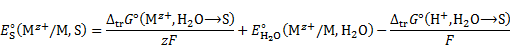##### Sections
You are here: Home Formulaes and Remarks relating to unified reducity
##### Document Actions

The PPM-Project was initially funded by_____________________________

UnipHied is funded byEURAMET

# Formulaes and Remarks relating to unified reducity

The defintion of the peabs is:The peabs can be calculated without the knowledge of the absolute chemical potential of the electron. The reason can be found by considering the Born-Fajans-Haber cycle (BFHC) of the H2-electrolysis where in equilibrium the electron can adopt any arbitrary state.From the BFHC follows that only the Standard Gibbs energy of solvation of the proton is needed to calculate the peabs of the system H+/H2:Since the first term contains the Standard Gibbs energies of formation and ionization of the hydrogen atom it is solvent independent. The second and third term are activity dependent terms whereby pHS is the pH value in the pH scale of the solvent S. For the calculation of pHS see “Formulas and remarks to pHabs”.

Other redox systems than H+/H2 demand their own BFHC resulting in individual but constant first terms and activity depending terms, e.g. for the system M+/M:Resulting in:where pKM is assigned to the Gibbs energy of formation and ionization, resp. In this way any redox system can be implemented into the peabs scale.

The peabs value can be converted into the conventional Volt scale via:With equation (4) one can determine the peabs via E°abs(Mz+/M,S) which is the redox potential of the system Mz+/M in the solvent S within the unified potential scale:with E°S(Mz+/M,S) is the redox potential of the system Mz+/M in the solvent S in the potential scale of S (i.e. vs. SHE in S) and E°abs(H+/H2,S) is the SHE in S within the unified potential scale. E°S(Mz+/M,S) can be obtained directly by the Gibbs energies of transfer:The zero point of the absolute pe scale peabs 0 is assigned to the ideal electron gas at 1 bar and 298.15 K. Here the chemical potential of the electron is 0 kJ mol−1.